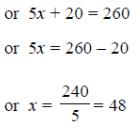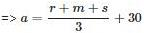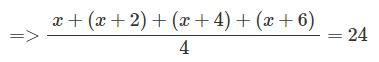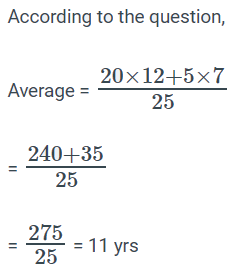Courses

# Average MCQ 1

## 20 Questions MCQ Test Quantitative Techniques for CLAT | Average MCQ 1

Description
This mock test of Average MCQ 1 for Quant helps you for every Quant entrance exam. This contains 20 Multiple Choice Questions for Quant Average MCQ 1 (mcq) to study with solutions a complete question bank. The solved questions answers in this Average MCQ 1 quiz give you a good mix of easy questions and tough questions. Quant students definitely take this Average MCQ 1 exercise for a better result in the exam. You can find other Average MCQ 1 extra questions, long questions & short questions for Quant on EduRev as well by searching above.
QUESTION: 1

### David obtained 76, 65, 82, 67 and 85 marks (out of 100) in English, Mathematics,Physics, Chemistry and Biology What are his average marks ?

Solution:

Average =   (76 + 65 + 82 + 67 + 85 )/ 5 = 375/5   =  75.

QUESTION: 2

### The average of 5 consecutive even numbers A, B, C, D and E is 52. What is the product of B and E?

Solution:

Let the five consecutive even numbers be x,
x + 2, x + 4, x + 6 and x + 8 respectively.
According to the question,
x + x + 2 + x + 4 + x + 6 + x + 8 = 5 × 52B = x + 2 = 48 + 2 = 50 and E = x + 8 = 48 + 8 = 56
B × E = 50 × 56 = 2800

QUESTION: 3

### The average weight of 8 persons increases by 2.5 kg when a new person comes in place of one of them weighing 65 kg. What might be the weight of the new person ?

Solution:

Total weight increased = (8 x 2.5) kg = 20 kg.

Weight of new person = (65 + 20) kg = 85 kg.

QUESTION: 4

Average score of Rahul, Manish & Suresh is 63. Rahul's score is 15 less than Ajay and 10 more than Manish. If Ajay scored 30 marks more than the average score of Rahul, Manish & Suresh, what is the sum of Manish's and Suresh's score?

Solution:

Let score of Rahul, Manish, Suresh, Ajay be r, m, s, a respectively
Average score of Rahul, Manish & Suresh is 63
⇒ Total score of Rahul, Manish & Suresh = 3 x 63 = 189
⇒ r + m + s = 189  —  (i)
Rahul's score is 15 less than Ajay and 10 more than Manish
r = a - 15 —(2)
r = m + 10 — (3)
Ajay scored 30 marks more than the average score of Rahul, Manish & Suresh⇒ 3a = r + m + s + 90 ----(4)
Using the value of r + m + s from (i) in (4)
3a = 189 + 90
a = 93
Using the value of a in (2)
r = 93 - 15
r = 78
Using the value of r in (i)
78 + m + 3 = 189
m + s = 111
i.e., sum of Manish's and Suresh's score = 111

QUESTION: 5

The average of four consecutive odd numbers is 24. Find the largest number.

Solution:

Let the numbers are x, x+2, x+4, x+6, then=>x+3=24=>x=21
So largest number is 21 + 6 = 27

So option B is correct answer.

QUESTION: 6

The average of five positive numbers is 308. The average of first two numbers is 482.5 and the average of last two numbers is 258.5. What is the third number.

Solution:

Let the numbers be A1, A2 , A3 , A4 , A5

► Average of 5 numbers = (A1 + A2 + A3 + A4 + A5) / 5 = 308

► Now A1 + A2 + A3 + A4 + A5 = 308 x 5 = 1540

► Also,A1 + A2 = 482.5 x 2 = 965

and A4 + A5 = 258.5 x 2 = 517

► A3 = 1540 − 965 − 517 = 58

QUESTION: 7

Average age of 54 girls in a class was found to be 10 years. It was later on realised that the actual age of one of the girls in the class was 8 years but it was wrongly taken as 18. The actual average age of the girls in the class is

Solution:

Summation of ages of 54 girls= 54*10= 540 since 18 is mistakely taken in place of 8 so total summation of ages of girls after correction= 540-18+8= 530 Now the actual average age of the girls = 530/54 =9.81

QUESTION: 8

The sum of seven consecutive numbers is 175. What is the sum of the first and the last number

Solution:

Sum of seven consecutive numbers x+(x+1)+(x+2)+(x+3)+(x+4)+(x+5)+(x+6)=175 7x+21=175
7x=154
x=22
First number will be 22 and consecutive series is 22, 23, 24, 25, 26, 27, 28

Sum of first and last number =22+28=50

QUESTION: 9

The average marks of nine students in a group is 63. Three of them scored 78, 69 and 48 marks. What are the average marks of remaining six students?

Solution:

The total marks of nine students = 9 × 63 = 567

Sum of the marks of three students = 78 + 69 + 48 = 195

Therefore, the sum of marks of the remaining six students= 567 – 195 = 372

Average marks of remaining six students = 372/6 = 62.

QUESTION: 10

The average age of 20 boys in a class is 12 years. 5 new boys are admitted to the class whose average age is 7 years. The average age of all the boys in the class becomes

Solution:QUESTION: 11

The average age of 24 boys and their teacher is 15 years. When the teacher’s age is excluded, the average age decreases by one year. The age of the teacher is

Solution:

Age of the teacher = (25×15−24×14)years
(375−336) years= 39years

QUESTION: 12

The average of five positive numbers is 213. The average of the first two numbers is 233.5 and the average of last two numbers is 271. What is the third number?

Solution:

► The sum of the five numbers = 5 * 213 =1065

► The sum of the first two numbers = 2 * 233.5 = 467

► The sum of  the last two numbers = 542

► Then the sum of the four numbers = 467 + 542 =1009

► So, the third number will be = 1065 – 1009 = 56

QUESTION: 13

The average weight of 8 men is increased by 1.5 kg when one of them whose weight is 65 kg is replaced by a new man. The weight of the new man is

Solution:

Total weight increased = (8 * 1.5) kg = 12 kg.
Weight of new man = 65 + 12 = 77 kg

QUESTION: 14

The average weight of 21 boys was recorded as 64 kg. If the weight of the teacher was added, the average increased by one kg. What was the teacher's weight?

Solution:

Here, n = 21, a = 64, b = 65
Weight of teacher = n (b - a) + b
= 21 (65 - 64) + 65 = 21 + 65 = 86 kg

QUESTION: 15

The average height of 16 students in a class is 142 cm. If the height of the teacher is added the average increases by 1 cm. What is the height of the teacher in cm?

Solution:

Height of the teacher = (17 * 143) − (16 * 142) = 2431 - 2272 = 159 cm

QUESTION: 16

The average weight of 15 girls was recorded as 54 kg. If the weight of the teacher was added, the average increased by two kg. What was the teacher's weight.

Solution:

Given that average is 54 kg for 15 girls.

(Sum of weight of 15 girls) / 15 = 54

Sum of weight of 15 girls = 15 x 54 = 810

Now weight of teacher is also added so the average becomes 54 + 1 kg = 55 kg

(Sum of weight of 15 girls + Weight of teacher ) / (15 girls + 1 teacher)  = New average = 55 (Given)

(810 + Weight of teacher ) / (16) = 55 + 1

810 + Weight of teacher = 16 (56)

Weight of teacher = 896 - 810 = 86 kg

QUESTION: 17

Find the average of first 10 even numbers

Solution:

"0" Is also an even number.
⇒ First 10 even numbers: 0, 2, 4, 6, 8, 10, 12, 14, 16, 18

Thus, average of first 10 even numbers = [ 0 + 2 + 4 + 6 + 8 + 10 + 12 + 14 + 16 + 18 ]  / 10 = 90/10 = 9

QUESTION: 18

Find the average of first 15 odd numbers

Solution:

► The first 15 odd numbers are 1,3,5,7,9,11,13,15,17,19,21,23,25,27,29

► The sum of first 15 odd numbers are 225

► Therefore mean is equal to 225/15=15

► Median =Middle value=15

QUESTION: 19

What will be the average of even numbers between 11 to 63

Solution:

Even numbers between 11 to 63
= 12,14,16,18,20,..........,62.
Clearly, this is a series of consecutive even numbers.

According to the formula,
Average of consecutive even numbers = (First number + Last number)/2 = (12 + 62)/2  = 74/2

QUESTION: 20

The average of 7 consecutive numbers is 20. The largest of these numbers is :

Solution:

Let the numbers be x, x + 1, x + 2,  x + 3, x + 4, x + 5 and x + 6
Then [x + (x + 1) + (x + 2) + (x + 3) + (x + 4) + (x + 5) + (x + 6)] / 7 = 20.
⇒ 7x + 21 = 140
⇒ 7x = 119
⇒ x =17.

Latest number = x + 6 = 23.